# AP Physics 2 Practice Test 18

### Test Information10 questions18 minutes

1.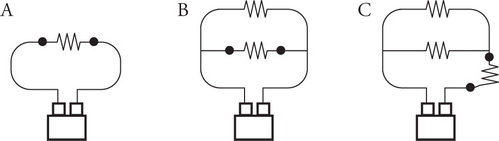Four identical batteries of negligible resistance are connected to resistors as shown. A voltmeter is connected to the points indicated by the dots in each circuit. Which of the following correctly ranks the potential difference measured by the voltmeter?

2.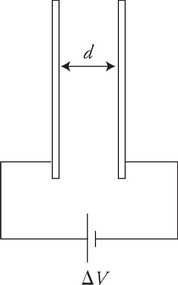A capacitor, with parallel plates a distance d apart, is connected to a battery of potential difference ΔV as shown in the figure. The plates of the capacitor can be moved inward or outward to change the distance d. To increase both the charge stored on the plates and the energy stored in the capacitor, which of the following should be done?

3.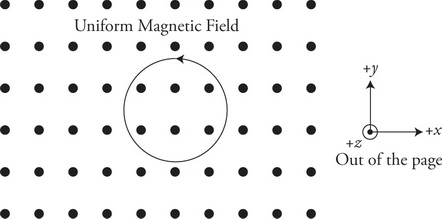A loop of wire with a counterclockwise current is immersed in a uniform magnetic field that is pointing up out of the paper in the +z direction. The loop is free to move in the field. Which of the following is a correct statement?

4.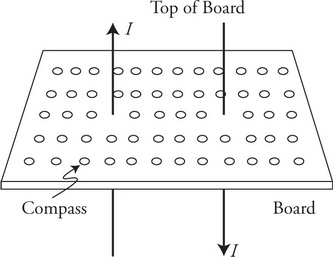Two long wires pass vertically through a horizontal board that is covered with an array of small compasses placed in a rectangular grid pattern as seen in the figure. The wire on the left has a current passing upward through the board, while the right wire has a current passing downward through the board. Both currents are identical in magnitude. Each compass has an arrow that points in the direction of north. Looking at the board from above, which of the following diagrams best depicts the directions that the array of compasses are pointing?

5.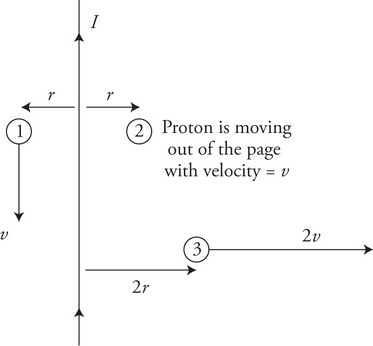A long wire carries a current as shown in the figure. Three protons are moving in the vicinity of the wire as shown. All three protons are in the plane of the page. Proton 1 in moving downward at a velocity of v. Proton 2 is moving out of the page at a velocity of v. Proton 3 is moving to the right at a velocity of 2v. Which of the following correctly ranks the magnetic force on the protons?

6.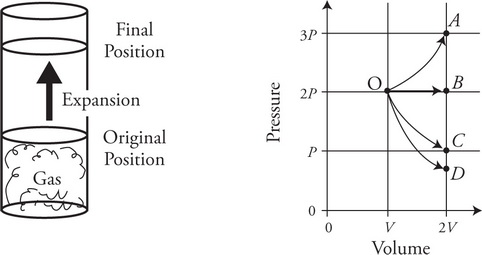A gas is confined in a sealed cylinder with a movable piston that is held in place as shown in the figure. The gas begins at an original volume V and pressure 2P, which is greater than atmospheric pressure. The piston is released and the gas expands to a final volume of 2 V. This expansion occurs very quickly such that there is very little heat transfer between the gas and the environment. Which of the following paths on the PV diagram best depicts this process?

7. An ideal gas is sealed in a fixed container. The container is placed in an oven, and the temperature of the gas is doubled. Which of the following correctly compares the final force the gas exerts on the container and the average speed of the molecules of the gas compared to the initial values?

Final Force on the Container from the Gas
Final Average Speed of Molecules

8. When hot water is poured into a beaker containing cold alcohol, the temperature of the mixture will eventually reach the same temperature. Considering the scale of the size of the molecules of the fluids, which of the following is the primary reason for this phenomenon?

9.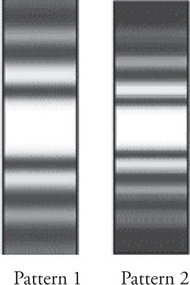In an experiment, monochromatic light of frequency f1 and wavelength λ1 passes through a single slit of width d1 to produce light and dark bands on a screen as seen in pattern 1. The screen is a distance L1 from the slit. A single change to the experimental setup is made and pattern 2 is created on the screen. Which of the following would account for the differences seen in the patterns?

10.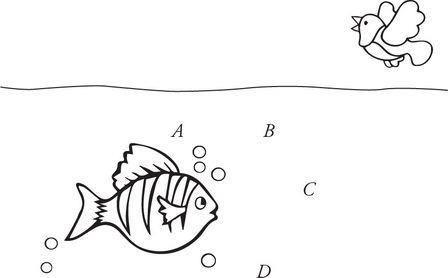A bird is flying over the ocean and sees a fish under the water. The actual positions of the bird and fish are shown in the figure. Assuming that the water is flat and calm, at which location does the bird perceive the fish to be?